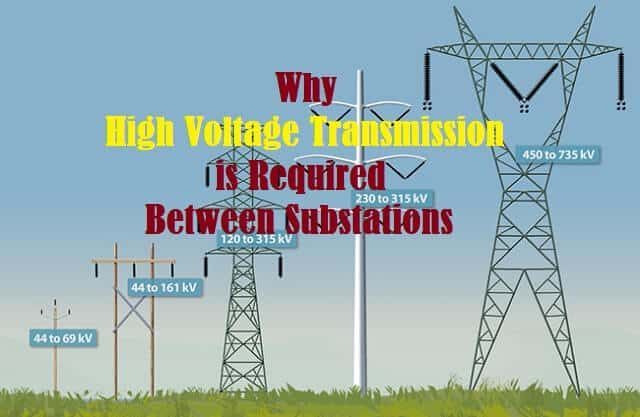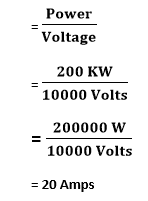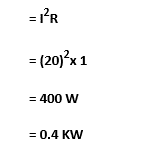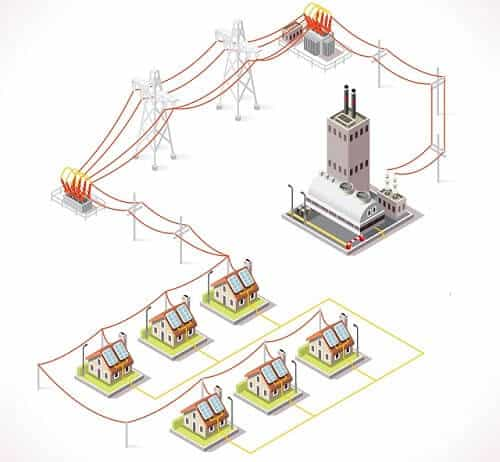Sunday, February 5, 2023
HomePower Generation & DistributionHigh Power TransmissionWhy High Voltage Transmission is Required Between Substations

# Why High Voltage Transmission is Required Between Substations

The Main Reason for High Voltage Transmission is to increase the efficiency and keep it economical. When transmission of Electricity is carried out over long distances, its happens along with voltage drop due to various losses involved along the path. With the help of High Voltage Transmission, we can reduce these losses as well as the costs involved in the conductor and hence improve the efficiency of power transmission and keep it economical.

Electrical Engineers around the world often consider aspects like voltage required to be transmitted to the end substation and the distance to be covered for calculating the optimal transmission voltage.

Now, to be more specific, let us understand it in detail.## Why High Voltage Transmission is Required

The network of Power Transmission must have effectiveness and safety compliance with cost efficiency. In order to comply with above requirements, Electric power transmission is carried out at high voltage to achieve following:

• Minimize the Power Losses.
• Minimize the cost in power transmission.

## Minimize Power Losses

The conductive path in a transmission line is generally made up of Aluminium (Al), Copper (Cu) or its alloys. It is the resistance of the conductor that has the maximum contribution in the Total Power Loss. The occurrence of loss in the Power lines because of the internal resistance of the conductor is Known as Copper Loss. The Copper loss is calculated as (Current)2 x Resistance i.e. I2R.

Accordingly, it may be stated that the Power Loss that happens in a transmission line is directly proportional to the current flowing through it. The higher the voltage will be the lesser will be the current and the lesser the current will be the lesser will be the power loss.

This is the reason, the line voltage is increased to reduce current (and eventually the Power loss) during Power transmission. If we will transmit the same power at a lower voltage to the similar distance, there will be more power loss.

Let us understand it better with some examples.

### Examples of How to Calculate Power Loss

Let us assume that we need to transmit 200KW of power to a distance of ‘X’ kms. The internal resistance of the conductor for the ‘X’ kms is 1 ohm. Now, we will calculate and compare the power loss happening here when the said power is transmitted at voltage levels of 1000 volts and 10000 volts.

#### Power Loss at 1000 Volts Transmission

As we know, Current through the conductor is:Therefore, Copper Loss in the transmission will be:#### Power Loss at 10000 Volts Transmission

Current through the conductor is:Therefore, Copper Loss in the transmission will be:#### Comparison of Power Loss at 1000v and 10000v Transmission

When the Power transmission is carried out at a lower voltage (1000v), the power loss is 40 KW whereas   when the Power transmission is carried out at a higher voltage (10000v), the power loss is 0.4 KW. The power loss during High Voltage transmission is much lower compared to low voltage transmission.

Therefore, it may be stated that we can reduce the power loss by transmitting the same power from a Power Grid to the end substation with High Voltage (power) Transmission. The reduction in losses minimizes the voltage drop and improves the voltage regulation.## Minimize the Cost in Power Transmission

The size of conductor in transmission line depends on the current range it has to carry. It is the current that defines what should be the cross section of the conducting line. If a said quantity of power is transmitting at a lower voltage level, the cross section of the conductor required will be larger than the the cross section of the conductor required for transmission of power at higher voltage level.

Therefore, the high voltage power transmission helps in reducing the quantity of conductor required. It needs no math that by reducing the quantity of conductor to be used, High Voltage Transmission reduces the cost involved.

```Also Read:
Hydroelectric Power Plant – Classification, Working & Applications
Underground Power Cables – Architecture, Applications and Advantages
Power Factor – Power Triangle, Types, PFC, Applications, Advantages```Ratna
Ratna is a B.E (Computer Science) and has work experience in UK Mainframe IT industry. She is also an active Web Designer. She is an author, editor and core partner at Electricalfundablog.
RELATED ARTICLES